# TD-CDFT Response properties for a 2D periodic system (NewResponse)¶

BAND can calculate response properties such as the frequency-dependent dielectric function within the theoretical framework of time-dependent current density function theory (TD-CDFT).

This introductory tutorial will show you how to:

• Set up and run a BAND single point calculation (using ADFJobs and ADFInput) for an MoS2 monolayer
• Set up and run a BAND TD-CDFT, linear response calculation (using ADFJobs and ADFInput) with the NewResponse method for an MoS2 monolayer
• Visualize the dielectric function using ADFSpectra

If you are not at all familiar with our Graphical User Interface (GUI), check out the Introductory tutorial first.

## Step 1: Create the system¶

We now want to create a MoS2 monolayer. Let us import the geometry from our database of structures

2. Switch from ADF to BAND.
3. Search for MoS2 in the search box (magnifying glass).
4. Select Crystals → MoS2_Monolayer. (you have to click on the triangle to the right of Crystals to expand the list)
5. click on View → Periodic → Repeat Unit CellsIf you succeed, your GUI should resemble the following picture: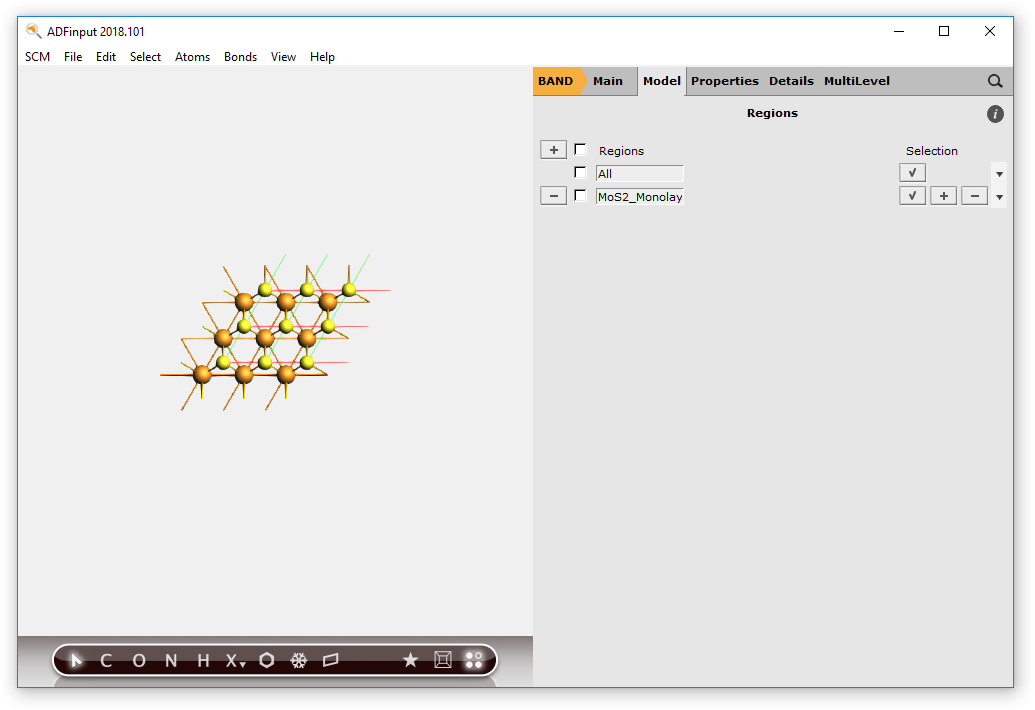## Step 2: Run a Singlepoint Calculations (LDA)¶

To get an idea regarding the performance of the LDA XC functional for the MoS2 monolayer, we first run a single-point calculation with the aim of analyzing the resulting band structure. We will already chose the calculation settings to be the same as for the following linear-response calculation with TD-CDFT: e.g. the basis set, the numerical quality, use of symmetry and the sampling of k-space.

1. Select the ‘BAND Main’ panel
2. Change Relativity to Scalar
3. Change Basis set to DZP
4. Change Numerical Quality to basic
5. Tick the Bandstructure and DOS check-boxes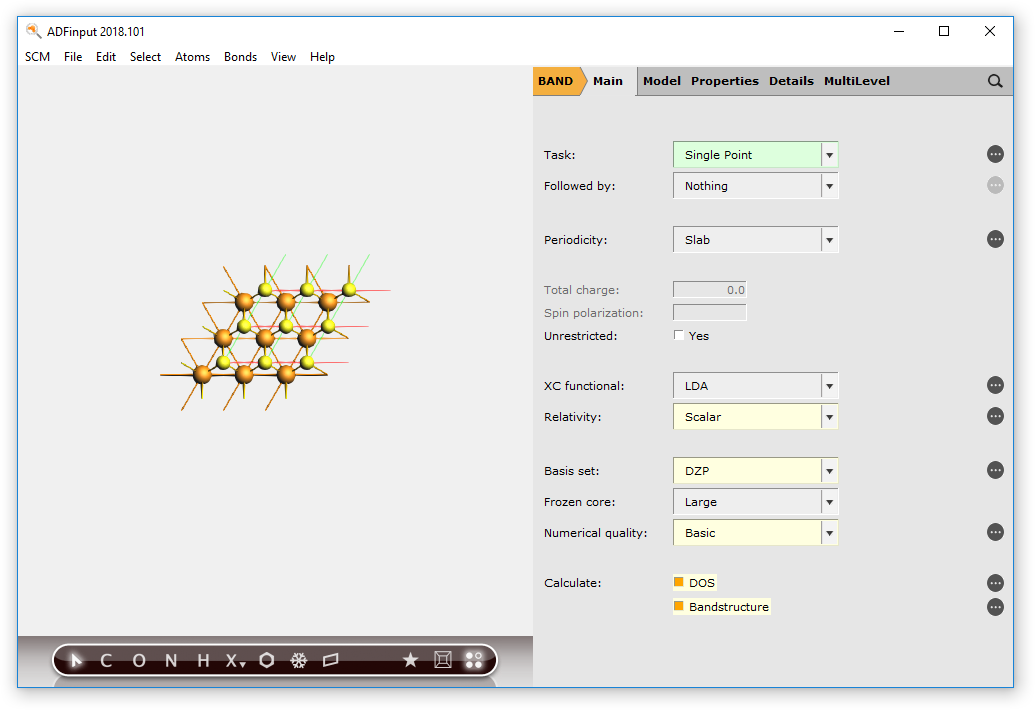6. Go to Details → K-Space Integration and set Number of points to ‘11 11‘.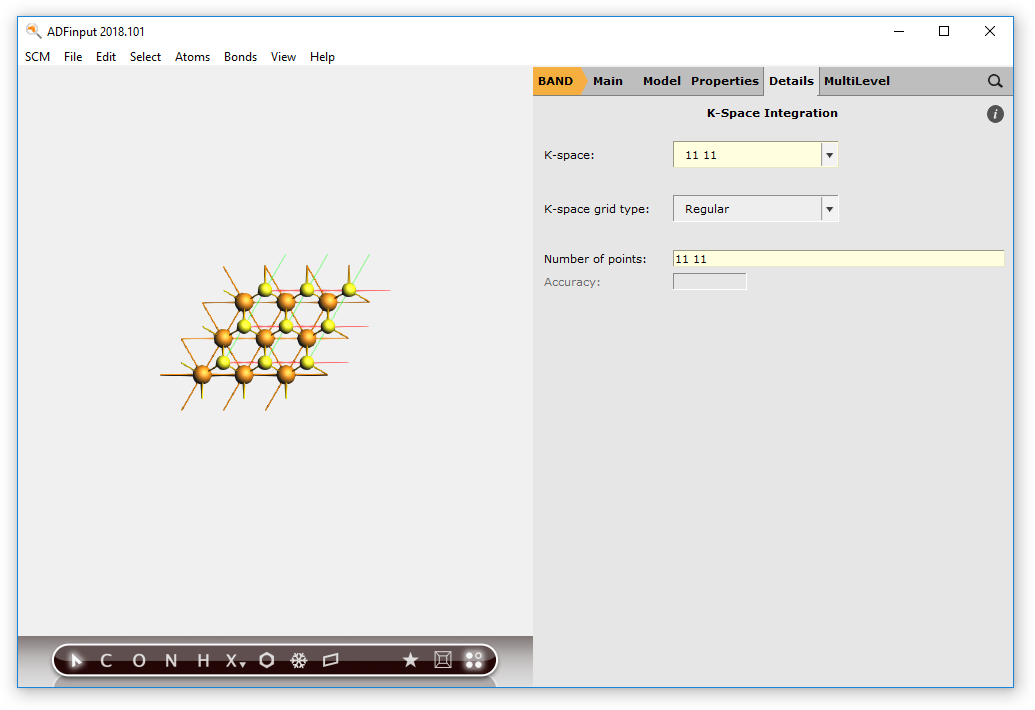7. Go to Details → Symmetry and de-select the check box Use of symmetry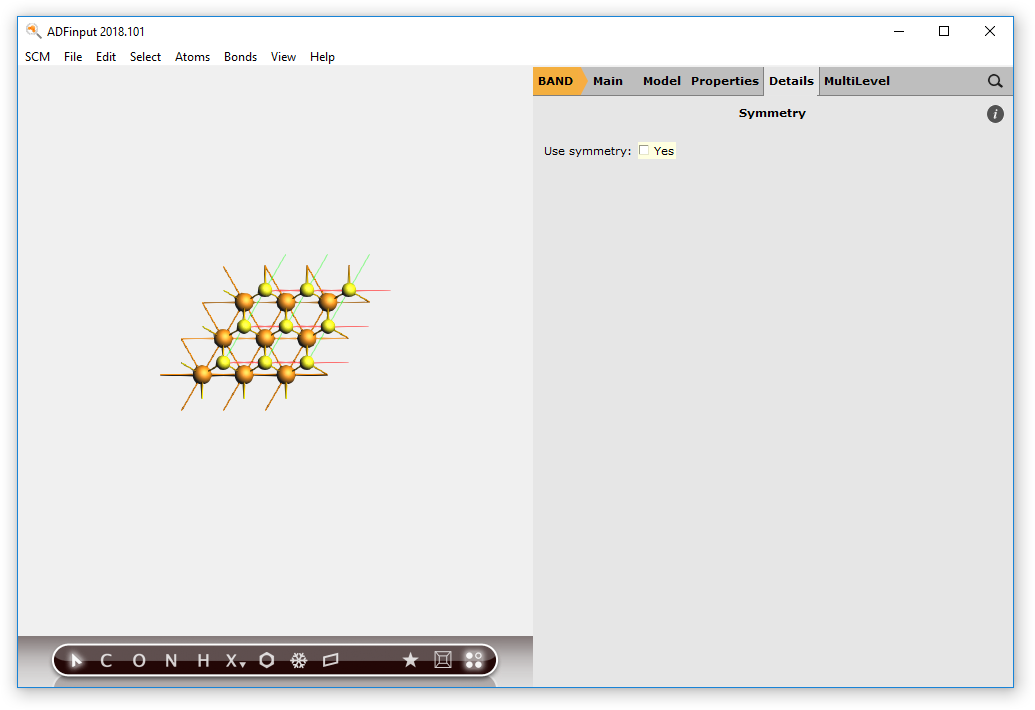Now, everything is prepared for the single-point calculation.

8. File → Save As..., use name LDA_SP.adf
9. File → Run

With the help of the bandstructure we can validate that basic electronic properties are reproduced by this k-space sampling using only LDA as XC functional. Unlike the multilayered MoS2, which has an indirect band gap, the MoS2 monolayer has a direct band-gap. (see also Ref)

10. SCM → Logfile
11. SCM → Bandstructure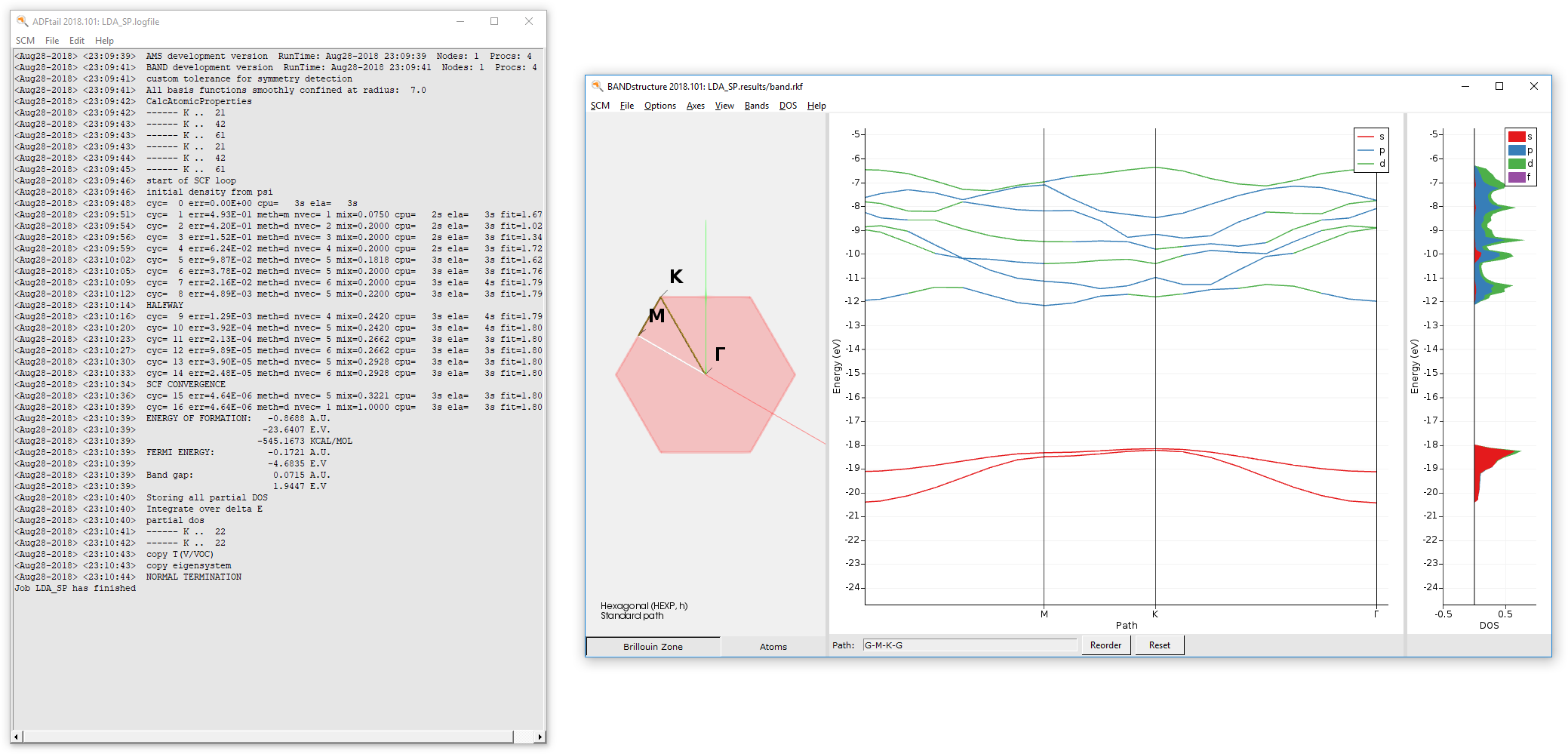## Step 3: Run an NewResponse Calculation (ALDA)¶

We can now start the calculation of the frequency-dependent, dielectric function using linear response TD-CDFT. As a reasonable frequency range we shall sample from 1.0 eV to 3.0 eV with a step size of 0.1 eV.

1. Go to the ‘BAND Main’ panel and uncheck the Bandstructure and DOS boxes.
2. Go to Properties → Dielectric Function (TD-CDFT).
3. Change Method to NewResponse.
4. Change Number of frequencies to 21.
5. Change Starting frequency to 1.0.
6. Change End frequency to 3.0.
7. Change Criterion to 0.1.
8. Uncheck the ‘z’-box regarding Components.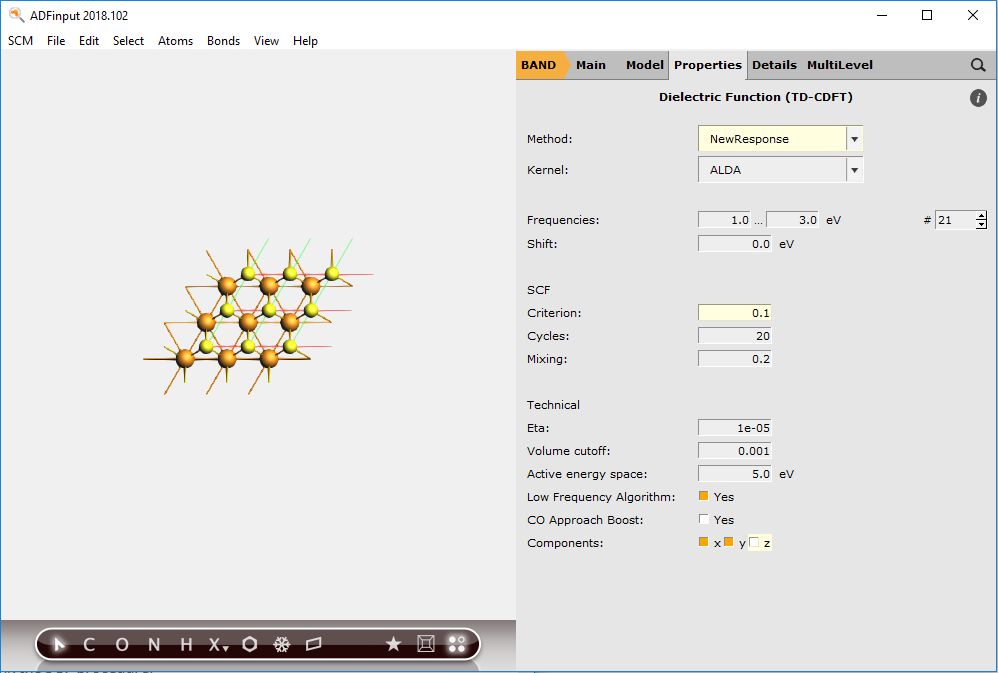Since we have already calculated the SCF results in the previous run, we can restart the SCF from the band.rkf file (this will save some time during the SCF procedure)

8. Go to Details → Restart.
9. Select the band.rkf file located in the folder LDA_SP.results
10. Tick the checkbox SCF next to Restart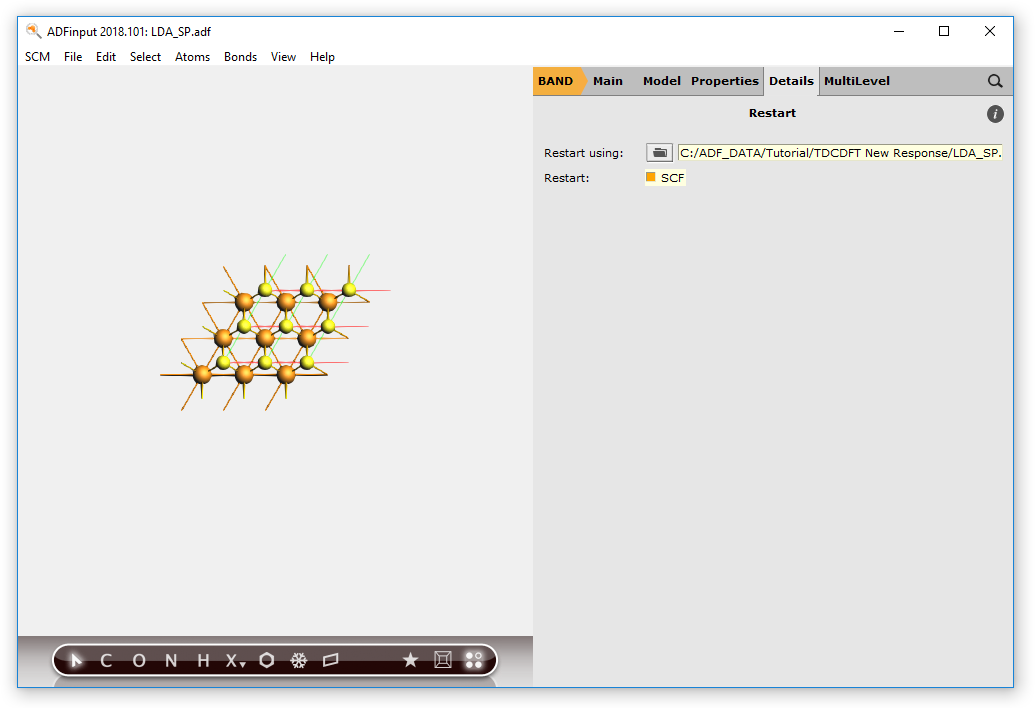Tip

This allows us to split the frequency range into smaller parts without too much of an overhead due to the SCF convergence for the ground state properties. One could even think about restarting from a hybrid DFT calculation with e.g. HSE06.

We are set to run the calculation now:

11. File → Save As..., use name ALDA_TDCDFT.adf
12. File → Run

After the calculation finished, we can analyze the calculated dielectric function by plotting it with ADFspectra.

13. SCM → Spectra
14. File → TD-CDFT → Dielectric Function → XXWith respect to the accuracy of our calculation we can reproduce the main features of the dielectric function for this system. We cannot expect the spin-orbit splitting of the first transition at around 1.9 eV to 2.1 eV, since we don’t use Spin-Orbit Relativistic ZORA. Furthermore, the absolute values of the dielectric function depends on the assumed two-dimensional volume for the surface. Since this definition is arbitrary, we can change the value for the Volume cutoff, so that the static dielectric function is reproduced.